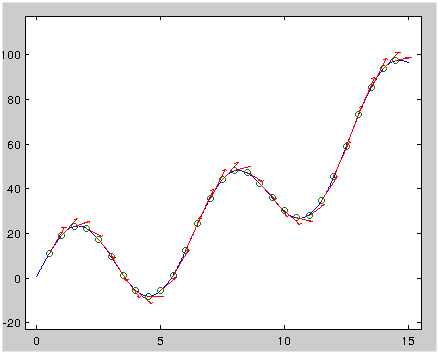## A special vector field made up of derivatives

A special vector field made up of derivatives

Make a vector field like this:

\begin{align*} F &= \begin{pmatrix} dy/dx \\ 1 \end{pmatrix} \end{align*}

It will show you the tangent lines.Vector field of tangent lines (matlab code)
Click to toggle code (may have to try twice)

x = 0:0.01:15 y = 20 .* sin(x) + .3 .* x.^2 + x + 1 x0 = .5:.5:14.5 y0 = 20 .* sin(x0) + .3 .* x0.^2 + x0 + 1 dy = 20 .* cos(x0) + .6 .* x0 + 1 dx = repmat(1, 1, size(dy, 2)) dy = dy * 10 dx = dx * 10 plot(x, y) hold on scatter(x0, y0) quiver(x0, y0, dx, dy, 'MaxHeadSize', .01)